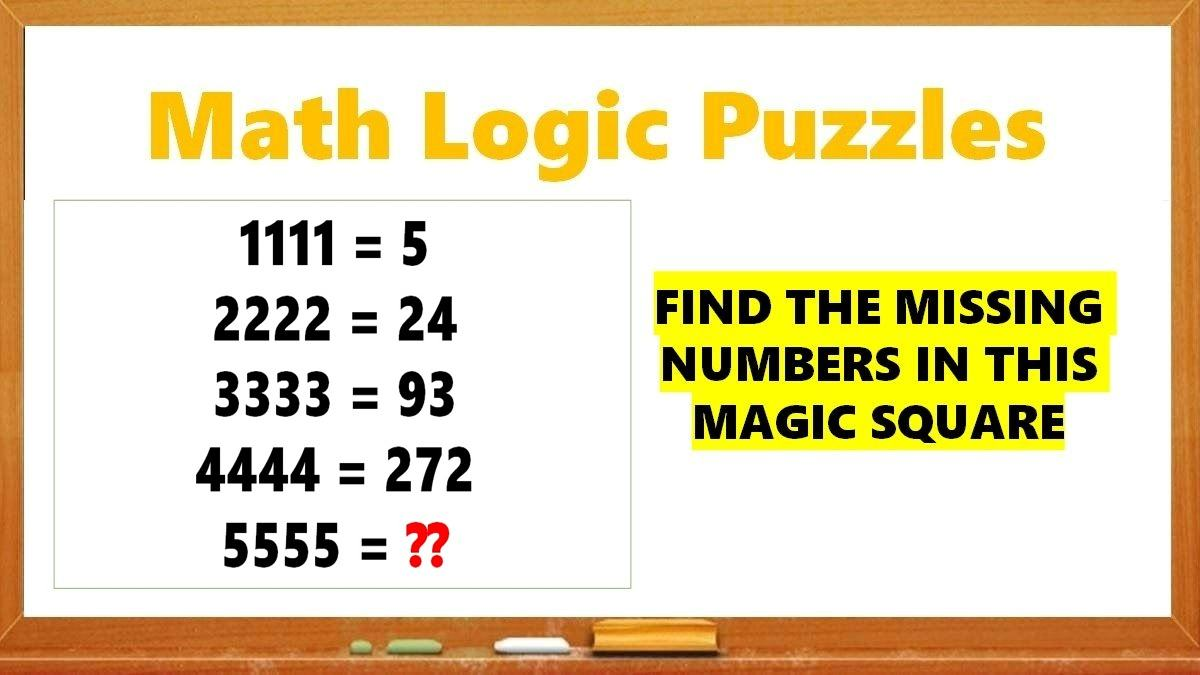# Math Riddles: Only 1 out 5 Geniuses Can Solve These Math Puzzles

Math Riddles: Check out this Math challenge. Can you solve these logic puzzles in 20 seconds each?Math Riddles: Only 1 out 5 Geniuses Can Solve These Math Puzzles

Math Riddles are so challenging, but that makes them worthwhile to solve. Math riddles are logical problems that require strong analytical abilities, high IQ, knowledge of math concepts, and good calculation skills. Math riddles have also been known to make Mathematics enjoyable for kids, students, and even adults to solve. Riddles, puzzles, and brain teasers can develop strategic thinking, logical reasoning, and problem-solving skills.

Today, we bring you another set of math puzzles that are all over social media. These Tricky Logic Math Puzzles have attracted millions of answers but very few got it right.

Can you solve this hard math magic square 3x3 puzzle? You have 20 seconds!

## Tricky Math Logic Puzzles Solution

Logic Puzzle #1

In each circle, the lower left value equals the sum of the numeric values of letters and top tight value equals the difference in the letter values. Assign 1 to 26 numbers to A to Z alphabets.

Let’s solve Circle #A to prove the theory.

Applying the logic as stated above, we get:

G = 7 and P = 16

=> G + P = 23

=> 7 + 16 = 23

And

=> P – G = 9

=> 16 – 7 = 9

Similarly, we will solve Circle #C

E = 5 and T = 20

=> E + T = 25

=> 5 + 20 = 25

And

=> T – E = ?

=> 20 – 5 = 15

Logic Puzzle #2

Answer: 14, 12, 16

We shall apply PEMDAS or BODMAS to solve these equations.

BODMAS is Bracket, Order, Division, Multiplication, Addition, and Subtraction.

Equation: 3 + 1 + 1 x 10 = ?

Hence, we will multiply first.

=> 3 + 1 + 10 = ?

=> 14

Equation: 3 + 3 x 3 – 3 + 3 = ?

Hence, we will multiply first again.

=> 3 + 9 – 3 + 3 = ?

=> 12 – 3 + 3 = ?

=> 15 – 3 = ?

=> 12

Equation: 8  2 (2 + 2) = ?

Hence, we will solve the bracket first then divide followed by multiplication.

=> 8  2 x 4 = ?

=> 4 x 4 = ?

=> 16

Logic Puzzle #3

1111 = 5

=> (1 + 1 + 1 + 1) + (1 x 1 x 1 x 1) = 5

2222 = 24

=> (2 + 2 + 2 + 2) + (2 x 2 x 2 x 2) = 24

3333 = 93

=> (3 + 3 + 3 + 3) + (3 x 3 x 3 x 3) = 93

4444 = 272

=> (4 + 4 + 4 + 4) + (4 x 4 x 4 x 4) = 272

Similarly,

5555 = ?

=> (5 + 5 + 5 + 5) + (5 x 5 x 5 x 5) = 645

Logic Puzzle #4

Notice, the numbers in the lower row are greater than the numbers in the upper row. The difference of top and bottom row numbers goes up by 3 with every box.

Now, ?? – 19 = 11 would mean ?? = 11 + 19 = 30.

Hence, missing number is 30.

Logic Puzzle #5

## Tell us in comments: Did you solve these logic puzzles correctly in 20 seconds?

Check out more math puzzles!

Also Read: Math Riddles: Solve This Magic Square Puzzle in 20 Seconds

Also Read: Math Riddles: Solve These Hard Logic Puzzles in 20 Seconds Each

Also Read: Math Riddles: Solve These Hard Logic Puzzles in 20 Seconds Each

Also Read: Math Riddles: Solve these Tricky Logic Puzzles in 20 seconds each

Also Read: Math Riddles: Find Missing Numbers in these Tricky Logic Math Puzzles

Also Read: Math Riddles with Answers: Can You Solve These Tricky Math Puzzles?

Get the latest General Knowledge and Current Affairs from all over India and world for all competitive exams.
खेलें हर किस्म के रोमांच से भरपूर गेम्स सिर्फ़ जागरण प्ले पर